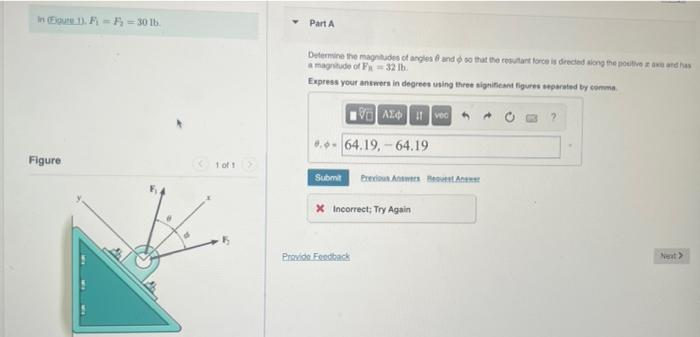# Question Solved1 Answer In (Eigure 1). F-F₁-30 lb. Figure 100 1 Part A Determine the magnitudes of angles and so that the resultant force is directed along the positive axi and has a magnitude of F = 32 lb. Express your answers in degrees using three significant figures separated by comma VAX i vec -64.19,-64.19 Submit Previous Answers Be X Incorrect; Try Again Next> Provide In (Eigure 1). F-F₁-30 lb. Figure 100 1 Part A Determine the magnitudes of angles and so that the resultant force is directed along the positive axi and has a magnitude of F = 32 lb. Express your answers in degrees using three significant figures separated by comma VAX i vec -64.19,-64.19 Submit Previous Answers Be X Incorrect; Try Again Next> Provide FeedbackFMHV4F The Asker · Civil EngineeringTranscribed Image Text: In (Eigure 1). F-F₁-30 lb. Figure 100 1 Part A Determine the magnitudes of angles and so that the resultant force is directed along the positive axi and has a magnitude of F = 32 lb. Express your answers in degrees using three significant figures separated by comma VAX i vec -64.19,-64.19 Submit Previous Answers Be X Incorrect; Try Again Next> Provide Feedback
More
Transcribed Image Text: In (Eigure 1). F-F₁-30 lb. Figure 100 1 Part A Determine the magnitudes of angles and so that the resultant force is directed along the positive axi and has a magnitude of F = 32 lb. Express your answers in degrees using three significant figures separated by comma VAX i vec -64.19,-64.19 Submit Previous Answers Be X Incorrect; Try Again Next> Provide Feedback